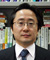# Artificial Intelligence and Society: Philosophy of FallibilityPart 14: Considering the Theory of Innovation-Driven Justice in Terms of Mathematical EquationsKOBAYASHI Keiichiro
Faculty Fellow, RIETI

If our argument is to be summarized in terms of mathematical equations, Formulas (1) to (3) shown in Part 8 apply. The symbol $$c_{t}$$ refers to individual activity conducted in the year $$t$$. The moral value of $$c_{t}$$ is expressed as $$p_{t}$$. The status of the Rawlsian social system in the year $$t$$ is expressed as $$k_{t}$$. The moral value of $$k_{t}$$ (=the status of the system) is expressed as $$q_{t}$$.

The meaning of Formula (1) is that the social system is valuable because it contributes to individual virtue. When the value of an individual life is expressed as $$p_{t}$$, if $$k_{t}$$ (=the status of the system) is to increase $$c_{t}$$ (= individual activity) by the multiplying ratio $$α$$, $$q_{t}$$ (=the value of the system) can be obtained through Formula (1).

$$q_{t}=αp_{t}$$ （1）
$$p_{t}=βq_{t+1}$$（2）
$$q_{t}=α×β×q_{t+1}＝γq_{t+1}$$（3）
(however, $$0<α<1, \:0<β<1, \:γ=α×β)$$
$$q_{t}=γq_{t+1}=γ^{2}q_{t+2}=γ^{3} q_{t+3}= … = γ^{N}×q_{t+N}$$（4）
$$q_{t} \lt q_{t+1} \lt q_{t+2} \lt q_{t+3}< … \lt q_{t+N} \lt q_{t+N+1} \lt …$$（5）

The meaning of Formula (2) is that individual virtue is valuable because it contributes to the social system. When the value of the social system $$q_{t}$$ has a given value, if it is assumed that individual activity improves the status of the system by the multiplying ratio $$β$$, the moral value $$p_{t}$$ of individual activity $$c_{t}$$ can be obtained under Formula 2. The time factor is included in this equation. More specifically, as the status of the system ($$k_{t}$$) in the current year (year $$t$$) is already fixed, individual activity in the current year ($$c_{t}$$) improves the status of the system in the next year ($$k_{t+1}$$) by the multiplying ratio $$β$$. Formula (3) is obtained by substituting the sign $$p_{t}$$ in Formula (1) with Formula (2).

Formula (3) expresses the following relationship. The status of the system ($$k_{t}$$) in the current year (the year $$t$$), expressed as $$q_{t}$$, is obtained by discounting the value of the system in the next year (the year $$t$$＋1), expressed as $$q_{t+1}$$, by the multiplying ratio $$γ$$.

Formula (3) continues to be valid beyond the next year. If Formula 3 is extended to future years, Formula (4) is obtained. The year $$t+N$$ represents an infinite future. In other words, the value of the system in the current year ($$q_{t}$$) depends on the value of the system in the next year ($$q_{t+1}$$), which in turn depends on the value of the system two years later ($$q_{t+2}$$,) and this relationship continues forever. If so, the value of the system in the current year($$q_{t}$$) ultimately depends on the value of the system in the future ($$q_{∞}$$). As the value of individual goods ($$p_{t}$$) also depends on $$q_{{t+1}}$$ , it is determined on the basis of the value of the social system in an infinite future.

$$γq_{t+N+1}=q_{t+N}=0$$（6）
$$q_{t+N-1}=γq_{t+N}=0$$（7）
$$q_{t+N-2}=γq_{t+N-1}=0$$（8）
$$q_t=q_{t+1}=q_{t+2}=…=q_{t+N}=0$$（9）
$$p_t=βq_{t+1}=0$$（10）

If reason has limits, that is, if innovation cannot last forever, such a chain of value cannot be realized. Let us assume that innovation will dry up at some point in the future (the year $$t + N$$) due to the limits on the progress of reason. In that case, under Formula (2), individual activity does not contribute to the updating of the social system, so it can be interpreted that $$β$$ is zero ($$β＝0$$) in the year $$t + N$$. As a result, the following equation is obtained: $$γ＝α×β＝0$$. If $$γ$$ is zero ($$γ＝0$$) in the year $$t + N$$ under Formula (4), $$γ$$ is zero ($$γ＝0$$) under Formula (6) as well, resulting in the equation $$q_{t+N}=0$$. In that case, even if $$γ$$ continues to be larger than zero ($$γ>0$$) until the year before the year $$t+N$$, $$q_{t+N-1}$$ is zero ($$q_{t+N-1}＝0$$) under Formula (7), while $$q_{t+N-2}$$ is zero $$q_{t+N-2}＝0$$) under Formula (8). If the time sequence of the chain of value is traced back in time as above, ultimately, Formula (9) applies, which means that $$q_{t}$$ is zero ($$q_{t}＝0$$). In other words, if innovation cannot last forever, the moral value of the social system at present (the year $$t$$) is zero, and consequently, the moral value of individual virtue $$p_{t}$$ is also zero at present under Formula (10).

Today, in the 21st century, humans have become able to believe that expanded reason through the power of artificial intelligence will continue forever, with the result that the creation of innovations by humans equipped with expanded reason will continue for a while without reaching any limit. That has made it possible for us to establish a "theory of innovation-driven justice" under which the perpetual chain of moral value represented by Formulas (1), (2) and (3) is realized. This is a moderate doctrine that comprehensively explains that individuals' lives have moral value, and that the social system also has value that should be preserved.

December 19, 2022

## Article(s) by this author

• ### Artificial Intelligence and Society: Philosophy of FallibilityPart 22: Fallibility and Freedom

August 21, 2023［Artificial Intelligence and Society: Philosophy of Fallibility］

• ### Intergenerational Problems Can Be Overcome

August 4, 2023［Newspapers & Magazines］

• ### Artificial Intelligence and Society: Philosophy of FallibilityPart 21: AI and Anti-Data Monopoly Policy

July 25, 2023［Artificial Intelligence and Society: Philosophy of Fallibility］

• ### Artificial Intelligence and Society: Philosophy of FallibilityPart 20: From the Quest for Infallibility to Fallibility

June 19, 2023［Artificial Intelligence and Society: Philosophy of Fallibility］

• ### Artificial Intelligence and Society: Philosophy of FallibilityPart 19: Innovation and a New School of Economics

May 22, 2023［Artificial Intelligence and Society: Philosophy of Fallibility］